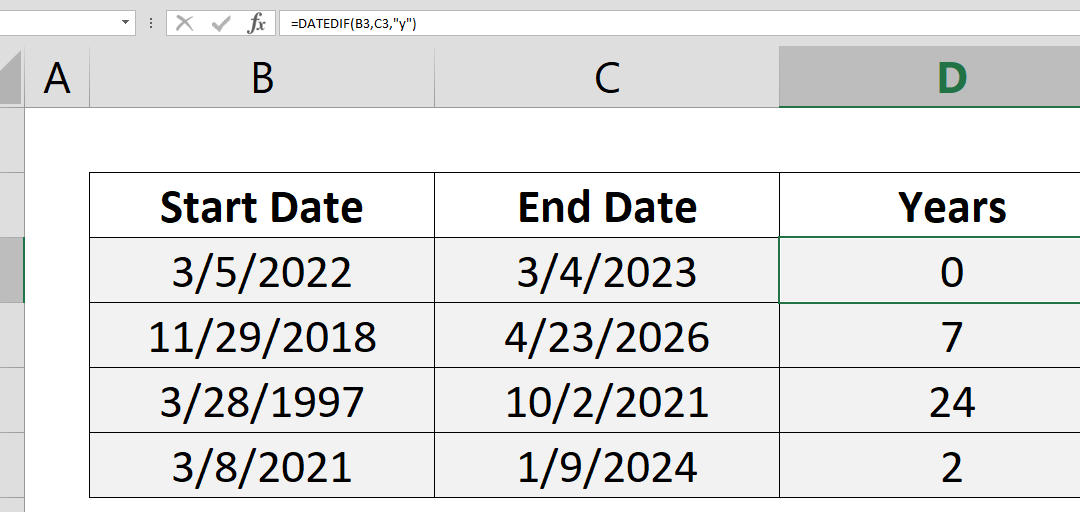Select Page## How to calculate Basic Tax Rate with VLOOKUP

The tax brackets can be confusing to work with. Different ‘levels’ of your income needs to be multiplied with a different percentage to find the total. This can be done with the conventional Excel formulas like IF and a bunch of others, but that’s the long and hard to...## How to check if a value exists in a list

The logical tests and conditional checks have important role in Excel models. Potential errors can be detected and handled by using the right logical tests. If you know How to check if a value exists in a list, you can use this logical statement to detect and...## How to find smallest value in array using a criteria

This article shows how to find smallest value in array using SMALL and IF functions in an array formula. Syntax {=SMALL(IF(criteria range = criteria, data range reference), nth)} Steps Start with =SMALL( function Continue with IF( Type the criteria range-criteria...## How to find largest value in array using a criteria

This article shows how to find largest value in array using LARGE and IF functions in an array formula. Syntax {=LARGE(IF(criteria range = criteria, data range reference), nth)} Steps Start with =LARGE( function Continue with IF( to apply condition Type the criteria...## How to find nth smallest value in a data table

This article shows you how to find nth smallest value in a data table by using the SMALL function. Syntax =SMALL(absolute data range reference, nth) Steps Start with =SMALL( function Select the range that contains the values \$B\$2:\$G\$7, Select the cell that contains...## How to find nth largest value in a data table

This article shows you that how to find nth largest value by using the LARGE function. Syntax =LARGE(absolute data range reference, nth) Steps Start with =LARGE( function Select the range that contains the values \$B\$2:\$G\$7, Select the cell that contains nth value or...## How to highlight cells by values

Highlighting cells is a good way to visualize content to locate areas that needs attention. Excel’s Conditional Formatting feature is a great tool for this job. How to highlight cells by values article will show how you can create highlight cells based on...## How to count values by length

This article shows how to count values by length based on their character count. This method can be helpful to check your data against invalid entries. It is also a good practice to use SUMPRODUCT and N functions together. Syntax =SUMPRODUCT(N(LEN(range)>character...## How to count years between two dates with the DATEDIF

This article show you how to count years between two dates using the DATEDIF function. Syntax =DATEDIF(start date, end date, “y”) Steps Use =DATEDIF( Select or type range reference that includes start date B3, Select or type range reference that includes...